xTime derivativeEncyclopedia
A time derivative is a derivative
Derivative
In calculus, a branch of mathematics, the derivative is a measure of how a function changes as its input changes. Loosely speaking, a derivative can be thought of as how much one quantity is changing in response to changes in some other quantity; for example, the derivative of the position of a...

of a function with respect to time
Time
Time is a part of the measuring system used to sequence events, to compare the durations of events and the intervals between them, and to quantify rates of change such as the motions of objects....

, usually interpreted as the rate of change
Derivative
In calculus, a branch of mathematics, the derivative is a measure of how a function changes as its input changes. Loosely speaking, a derivative can be thought of as how much one quantity is changing in response to changes in some other quantity; for example, the derivative of the position of a...

of the value of the function. The variable denoting time is usually written as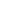.

## Notation

A variety of notations are used to denote the time derivative. In addition to the normal (Leibniz's) notation,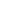two very common shorthand notations are also used: adding a dot over the variable,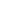(Newton's notation), and adding a prime
Prime (symbol)
The prime symbol , double prime symbol , and triple prime symbol , etc., are used to designate several different units, and for various other purposes in mathematics, the sciences and linguistics...

to the function,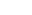(Lagrange's notation). These two shorthands are generally not mixed in the same set of equations.

Higher time derivatives are also used: the second derivative
Second derivative
In calculus, the second derivative of a function ƒ is the derivative of the derivative of ƒ. Roughly speaking, the second derivative measures how the rate of change of a quantity is itself changing; for example, the second derivative of the position of a vehicle with respect to time is...

with respect to time is written as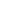with the corresponding shorthands of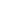and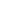.

As a generalization, the time derivative of a vector, say: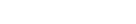is defined as the vector whose components are the derivatives of the components of the original vector. That is,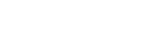## Use in physics

Time derivatives are a key concept in physics
Physics
Physics is a natural science that involves the study of matter and its motion through spacetime, along with related concepts such as energy and force. More broadly, it is the general analysis of nature, conducted in order to understand how the universe behaves.Physics is one of the oldest academic...

. For example, for a changing position, its time derivativeis its velocity
Velocity
In physics, velocity is speed in a given direction. Speed describes only how fast an object is moving, whereas velocity gives both the speed and direction of the object's motion. To have a constant velocity, an object must have a constant speed and motion in a constant direction. Constant ...

, and its second derivative with respect to time,, is its acceleration
Acceleration
In physics, acceleration is the rate of change of velocity with time. In one dimension, acceleration is the rate at which something speeds up or slows down. However, since velocity is a vector, acceleration describes the rate of change of both the magnitude and the direction of velocity. ...

. Even higher derivatives are sometimes also used: the third derivative of position with respect to time is known as the jerk. See motion graphs and derivatives
Motion graphs and derivatives
In mechanics, the derivative of the position vs. time graph of an object is equal to the velocity of the object. In the International System of Units, the position of the moving object is measured in meters relative to the origin, while the time is measured in seconds...

.

A large number of fundamental equations in physics involve first or second time derivatives of quantities. Many other fundamental quantities in science are time derivatives of one another:
• force
Force
In physics, a force is any influence that causes an object to undergo a change in speed, a change in direction, or a change in shape. In other words, a force is that which can cause an object with mass to change its velocity , i.e., to accelerate, or which can cause a flexible object to deform...

is the time derivative of momentum
Momentum
In classical mechanics, linear momentum or translational momentum is the product of the mass and velocity of an object...

• power
Power (physics)
In physics, power is the rate at which energy is transferred, used, or transformed. For example, the rate at which a light bulb transforms electrical energy into heat and light is measured in watts—the more wattage, the more power, or equivalently the more electrical energy is used per unit...

is the time derivative of energy
Energy
In physics, energy is an indirectly observed quantity. It is often understood as the ability a physical system has to do work on other physical systems...

• electrical current is the time derivative of electric charge
Electric charge
Electric charge is a physical property of matter that causes it to experience a force when near other electrically charged matter. Electric charge comes in two types, called positive and negative. Two positively charged substances, or objects, experience a mutual repulsive force, as do two...

and so on.

A common occurrence in physics is the time derivative of a vector, such as velocity or displacement. In dealing with such a derivative, both magnitude and orientation may depend upon time.

### Example: circular motion

For example, consider a particle moving in a circular path. Its position is given by the displacement vector , related to the angle, θ, and radial distance, ρ, as defined in Figure 1: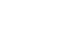For purposes of this example, time dependence is introduced by setting . The displacement (position) at any time t is then: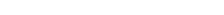The second form shows the motion described by r(t) is in a circle of radius ρ because the magnitude of r(t) is given by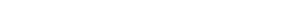using the trigonometric identity .

With this form for the displacement, the velocity now is found. The time derivative of the displacement vector is the velocity vector. In general, the derivative of a vector is a vector made up of components each of which is the derivative of the corresponding component of the original vector. Thus, in this case, the velocity vector is: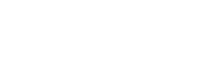Thus the velocity of the particle is nonzero even though the magnitude of the position (that is, the radius of the path) is constant. The velocity is directed perpendicular to the displacement, as can be established using the dot product
Dot product
In mathematics, the dot product or scalar product is an algebraic operation that takes two equal-length sequences of numbers and returns a single number obtained by multiplying corresponding entries and then summing those products...

: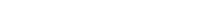Acceleration is then the time-derivative of velocity: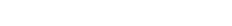The acceleration is directed inward, toward the axis of rotation. It points opposite to the position vector and perpendicular to the velocity vector. This inward-directed acceleration is called centripetal acceleration
Centripetal force
Centripetal force is a force that makes a body follow a curved path: it is always directed orthogonal to the velocity of the body, toward the instantaneous center of curvature of the path. The mathematical description was derived in 1659 by Dutch physicist Christiaan Huygens...

.

## Use in economics

In economics
Economics
Economics is the social science that analyzes the production, distribution, and consumption of goods and services. The term economics comes from the Ancient Greek from + , hence "rules of the house"...

, many theoretical models of the evolution of various economic variables are constructed in continuous time and therefore employ time derivatives. See for example exogenous growth model
Exogenous growth model
The neoclassical growth model, also known as the Solow–Swan growth model or exogenous growth model, is a class of economic models of long-run economic growth set within the framework of neoclassical economics...

and ch. 1-3. One situation involves a stock variable and its time derivative, a flow variable. Examples include:
• The flow of net fixed investment
Fixed investment
Fixed investment in economics refers to investment in fixed capital, i.e., tangible capital goods , or to the replacement of depreciated capital goods which have been scrapped....

is the time derivative of the capital stock.
• The flow of inventory investment
Inventory investment
Inventory investment is a component of gross domestic product . What is produced in a certain country is naturally also sold eventually, but some of the goods produced in a given year may be sold in a later year rather than in the year they were produced. Conversely, some of the goods sold in a...

is the time derivative of the stock of inventories.
• The growth rate of the money supply
Money supply
In economics, the money supply or money stock, is the total amount of money available in an economy at a specific time. There are several ways to define "money," but standard measures usually include currency in circulation and demand deposits .Money supply data are recorded and published, usually...

is the time derivative of the money supply divided by the money supply itself.

Sometimes the time derivative of a flow variable can appear in a model:
• The growth rate of output
Output
Output is the term denoting either an exit or changes which exit a system and which activate/modify a process. It is an abstract concept, used in the modeling, system design and system exploitation.-In control theory:...

is the time derivative of the flow of output divided by output itself.
• The growth rate of the labor force
Labor force
In economics, a labor force or labour force is a region's combined civilian workforce, including both the employed and unemployed.Normally, the labor force of a country consists of everyone of working age In economics, a labor force or labour force is a region's combined civilian workforce,...

is the time derivative of the labor force divided by the labor force itself.

And sometimes there appears a time derivative of a variable which, unlike the examples above, is not measured in units of currency:
• The time derivative of a key interest rate
Interest rate
An interest rate is the rate at which interest is paid by a borrower for the use of money that they borrow from a lender. For example, a small company borrows capital from a bank to buy new assets for their business, and in return the lender receives interest at a predetermined interest rate for...

can appear.
• The inflation rate
Inflation rate
In economics, the inflation rate is a measure of inflation, the rate of increase of a price index . It is the percentage rate of change in price level over time. The rate of decrease in the purchasing power of money is approximately equal.The inflation rate is used to calculate the real interest...

is the growth rate of the price level
Price level
A price level is a hypothetical measure of overall prices for some set of goods and services, in a given region during a given interval, normalized relative to some base set...

—that is, the time derivative of the price level divided by the price level itself.

• Differential calculus
Differential calculus
In mathematics, differential calculus is a subfield of calculus concerned with the study of the rates at which quantities change. It is one of the two traditional divisions of calculus, the other being integral calculus....

• Notation for differentiation
Notation for differentiation
In differential calculus, there is no single uniform notation for differentiation. Instead, several different notations for the derivative of a function or variable have been proposed by different mathematicians. The usefulness of each notation varies with the context, and it is sometimes...

• Circular motion
Circular motion
In physics, circular motion is rotation along a circular path or a circular orbit. It can be uniform, that is, with constant angular rate of rotation , or non-uniform, that is, with a changing rate of rotation. The rotation around a fixed axis of a three-dimensional body involves circular motion of...

• Centripetal force
Centripetal force
Centripetal force is a force that makes a body follow a curved path: it is always directed orthogonal to the velocity of the body, toward the instantaneous center of curvature of the path. The mathematical description was derived in 1659 by Dutch physicist Christiaan Huygens...